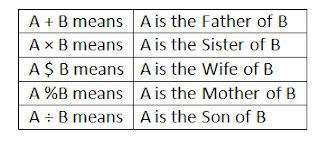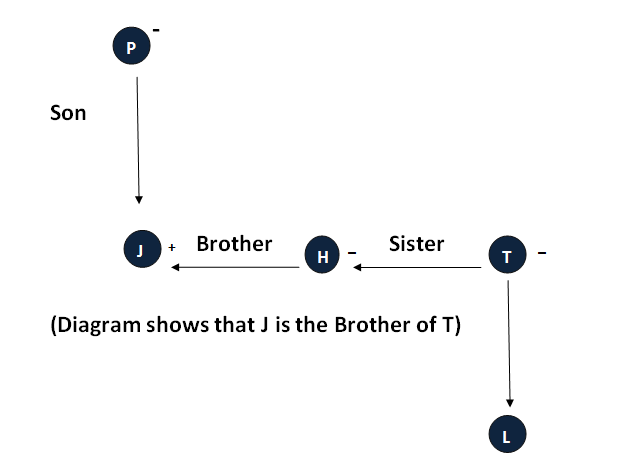# Blood Relation Test - Question and Answer

Q. (1 - 5) Read the information carefully and answer the questions:Q1. What should come in place of the question mark, to establish that J is the brother of T in the expression = J ÷ P % H ? T % L
a) ×
b) ÷

c) \$
e) None of The Above

Q2. Which among the given expression indicate that M is the daughter of D:
a) L + R \$ D × M × T
b) L \$ R \$ D + M × T
c) L + R \$ D + M × T
d) None of The Above

Q3. Which among the following options is true, if the expression I + T % J × L ÷ K is definitely true:
a) L is the daughter of T
b) K is the son - in - law of I
c) I is the grandmother of L
d) J is the brother of L
e) None of The Above

Q4. Which among the following expressions is true, if Y is the son of X is definitely false:
a) W % L × T × Y ÷ X
b) W \$ X + L + Y + T
c) W % X + T × Y ÷ L
d) None of The Above

Q5. What should come in place of the question mark, to establish that T is the sister - in - law of Q in the expression = R % T × P ? Q + V
a) +
b) ×
c) \$
d) None of The Above

### Solutions